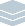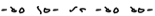Wolfram Archive
Wolfram Programming Lab is a legacy product.
All the same functionality and features, including access to Programming Lab Explorations, are available with Wolfram|One.
Start programming now. »# Random Alphabet

Make a random sequence of glyphs to serve as letters in an alphabet.

Run the code to draw a random line joining 4 points. Try numbers of points other than 4for example, 3:

This draws a line through the points at coordinates {0,0}, {1,1} and {2,0}:

Graphics[Line[{{0, 0}, {1, 1}, {2, 0}}]]

You can turn on axes with AxesTrue in order to see the numeric coordinate positions:

Graphics[Line[{{0, 0}, {1, 1}, {2, 0}}], Axes -> True]

Use RandomReal to draw a line with random coordinates.

This gives a random number between 0 and 1. Each time you run the code, you get a different number:

RandomReal

This gives a pair of numbers between 0 and 1. Each time you run the code, you get a different pair:

RandomReal[1, 2]

This gives four pairs of random numbers:

RandomReal[1, {4, 2}]

Use that code to draw a line with random coordinates:

Graphics[Line[RandomReal[1, {4, 2}]]]

Specify Thick to draw a thicker random line:

Graphics[{Thick, Line[RandomReal[1, {4, 2}]]}]

Graphics[{Thick, Line[RandomReal[1, {4, 2}]]}]

Draw a random line joining between 3 and 6 points. Try ranges other than {3,6}for example, {2,3}:

RandomReal gives random decimal numbers. For example, this gives a random decimal number between 3 and 6:

RandomReal[{3, 6}]

RandomInteger is like RandomReal, but gives only integers. This gives a random integer between 3 and 6:

RandomInteger[{3, 6}]

Recall that this draws random lines with 4 points:

Graphics[{Thick, Line[RandomReal[1, {4, 2}]]}]

Replace 4 with RandomInteger[{3,6}] to draw random lines with between 3 and 6 points:

Graphics[{Thick, Line[RandomReal[1, {RandomInteger[{3, 6}], 2}]]}]

Graphics[{Thick, Line[RandomReal[1, {RandomInteger[{3, 6}], 2}]]}]

Draw small, thick glyphs that could be characters in an alphabet. Try thicknesses other than 5:

This draws graphics at the default size:

Graphics[{Thick, Line[RandomReal[1, {RandomInteger[{3, 6}], 2}]]}]

Make a small 30×30 pixel graphic by specifying ImageSize{30,30}:

Graphics[{Thick, Line[RandomReal[1, {RandomInteger[{3, 6}], 2}]]}, ImageSize -> {30, 30}]

Replace Thick with AbsoluteThickness to get lines that are exactly 5 pixels thick:

Graphics[{AbsoluteThickness, Line[RandomReal[1, {RandomInteger[{3, 6}], 2}]]}, ImageSize -> {30, 30}]

Graphics[{AbsoluteThickness, Line[RandomReal[1, {RandomInteger[{3, 6}], 2}]]}, ImageSize -> {30, 30}]

Make a list of 26 random glyphs, each made by joining between 3 and 6 points. Try other numbers of glyphs:

Table makes lists of things. This uses Table to make a list of 26 random numbers:

Table[RandomReal[], {26}]

Replace RandomReal[] with the glyph code from the previous step to make a list of 26 random glyphs:

Table[Graphics[{AbsoluteThickness, Line[RandomReal[1, {RandomInteger[{3, 6}], 2}]]}, ImageSize -> {30, 30}], {26}]

Table[Graphics[{AbsoluteThickness, Line[RandomReal[1, {RandomInteger[{3, 6}], 2}]]}, ImageSize -> {30, 30}], {26}]

Make rounded glyphs that look more like cursive writing using B-spline curves:

This draws a line through several points:

Graphics[Line[{{0, 0}, {1, 0}, {1, 1}, {0, 1}}]]

If you replace Line with BSplineCurve, you get a smooth curve that approximates the path of the line:

Graphics[BSplineCurve[{{0, 0}, {1, 0}, {1, 1}, {0, 1}}]]

Here are the line and the B-spline curve drawn in the same graphic so you can see how they correspond:

Graphics[{ Line[{{0, 0}, {1, 0}, {1, 1}, {0, 1}}], BSplineCurve[{{0, 0}, {1, 0}, {1, 1}, {0, 1}}] }]

Replace Line with BSplineCurve in the glyph expression to get smooth glyphs that look more like cursive writing:

Table[Graphics[{AbsoluteThickness, BSplineCurve[RandomReal[1, {RandomInteger[{3, 6}], 2}]]}, ImageSize -> {30, 30}], {26}]

Table[Graphics[{AbsoluteThickness, BSplineCurve[RandomReal[1, {RandomInteger[{3, 6}], 2}]]}, ImageSize -> {30, 30}], {26}]

Make rules that translate from the alphabet to glyphs:

With a set of rules that specifies which glyphs correspond to which letters, you can translate from words written with the normal alphabet to words written with glyphs. Each rule looks something like this:

"A" -> \!\(\* GraphicsBox[ {AbsoluteThickness, BSplineCurveBox[{{0.4987640325753826, 0.03522112143894818}, { 0.010385474885092894`, 0.506093796370255}, {0.2031651297296817, 0.27707917882476796`}, {0.7794878961873593, 0.07259744469065543}, {0.2202419352025109, 0.7526782436876707}, {0.9267225956920517, 0.8457783797241891}}]}, ImageSize->{30, 30}]\)

This gives the 26 capital letters in the alphabet. Give it the name letters:

letters = CharacterRange["A", "Z"]

This expression from the previous step gives 26 random glyphs. Give it the name glyphs:

glyphs = Table[ Graphics[{AbsoluteThickness, BSplineCurve[RandomReal[1, {RandomInteger[{3, 6}], 2}]]}, ImageSize -> {30, 30}], {26}]

Make rules from the letters to the glyphs using Thread. Thread changes a rule between lists to a list of rules between the elements of the lists:

Thread[{"A", "B", "C"} -> {1, 2, 3}]

This gives the translation rules from letters to glyphs:

We need one more rule to translate spaces to empty glyphs:

" " -> Graphics[ImageSize -> {30, 30}]

Append that rule to the other rules to get a complete set of translation rules. Call it alphabet:

alphabet = Append[Thread[letters -> glyphs], " " -> Graphics[ImageSize -> {30, 30}]]

letters = CharacterRange["A", "Z"]; glyphs = Table[ Graphics[{AbsoluteThickness, BSplineCurve[RandomReal[1, {RandomInteger[{3, 6}], 2}]]}, ImageSize -> {30, 30}], {26}]; alphabet = Append[Thread[letters -> glyphs], " " -> Graphics[ImageSize -> {30, 30}]]

Write out a sentence in the glyph alphabet. Try other sentences:

Note: make sure you run the code in the previous step first.

The translation rules in alphabet only work on uppercase letters, so the first step in translating a sentence to glyphs is to use ToUpperCase to make the letters in the sentence uppercase:

ToUpperCase["My name is wolfie"]

Then convert the uppercase text to a list of individual characters using Characters:

Characters[ToUpperCase["My name is wolfie"]]

Now you can translate the letters to glyphs using the translation rules in alphabet.

Heres an example of using rules to replace parts of an expression. Every 0 in the expression to the left of /. is replaced with an a and every 1 is replaced with a Z:

{0, 1, 0, 1, 1} /. {0 -> "a", 1 -> "Z"}

Applying the alphabet rules to the characters in the text translates the text to glyphs:

Characters[ToUpperCase["My name is wolfie"]] /. alphabet

Use Row to format the list of glyphs without commas and braces:

Row[Characters[ToUpperCase["My name is wolfie"]] /. alphabet]

Row[Characters[ToUpperCase["My name is wolfie"]] /. alphabet]

Write the beginning of the US Constitution in the glyph alphabet:

The alphabet rules are completely general and can be applied to any textfor example, the US Constitution.

This gives the first 200 characters of the text of the US Constitution:

constitution = StringTake[ExampleData[{"Text", "USConstitution"}], 200]

This translates the text to glyphs:

Row[Characters[ToUpperCase[constitution]] /. alphabet]

constitution = StringTake[ExampleData[{"Text", "USConstitution"}], 200]; Row[Characters[ToUpperCase[constitution]] /. alphabet]

Share Itmake a website that writes a text with a random alphabet:

Deploy a form that writes text that you enter using a random alphabet:

CloudDeploy[ FormFunction[{"text" -> "String"}, Block[{letters, glyphs, alphabet}, letters = CharacterRange["A", "Z"]; glyphs = Table[Graphics[{AbsoluteThickness, BSplineCurve[RandomReal[1, {RandomInteger[{3, 6}], 2}]]}, ImageSize -> {30, 30}], {26}]; alphabet = Append[Thread[letters -> glyphs], " " -> Graphics[ImageSize -> {30, 30}]]; Row[Characters[ToUpperCase[#text]] /. alphabet] ] &, "PNG" ], Permissions -> "Public" ]

Click the link in the output to visit the site.